Learnyst Support

# How To Add Math Equations Within Your Quiz - E.g: Fractions, Powers, Symbols

Would you like to insert a math equation in your quiz? For example like this question below.

Q.1) Find the nature of the below quadratic equation. If the real roots exist, find them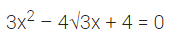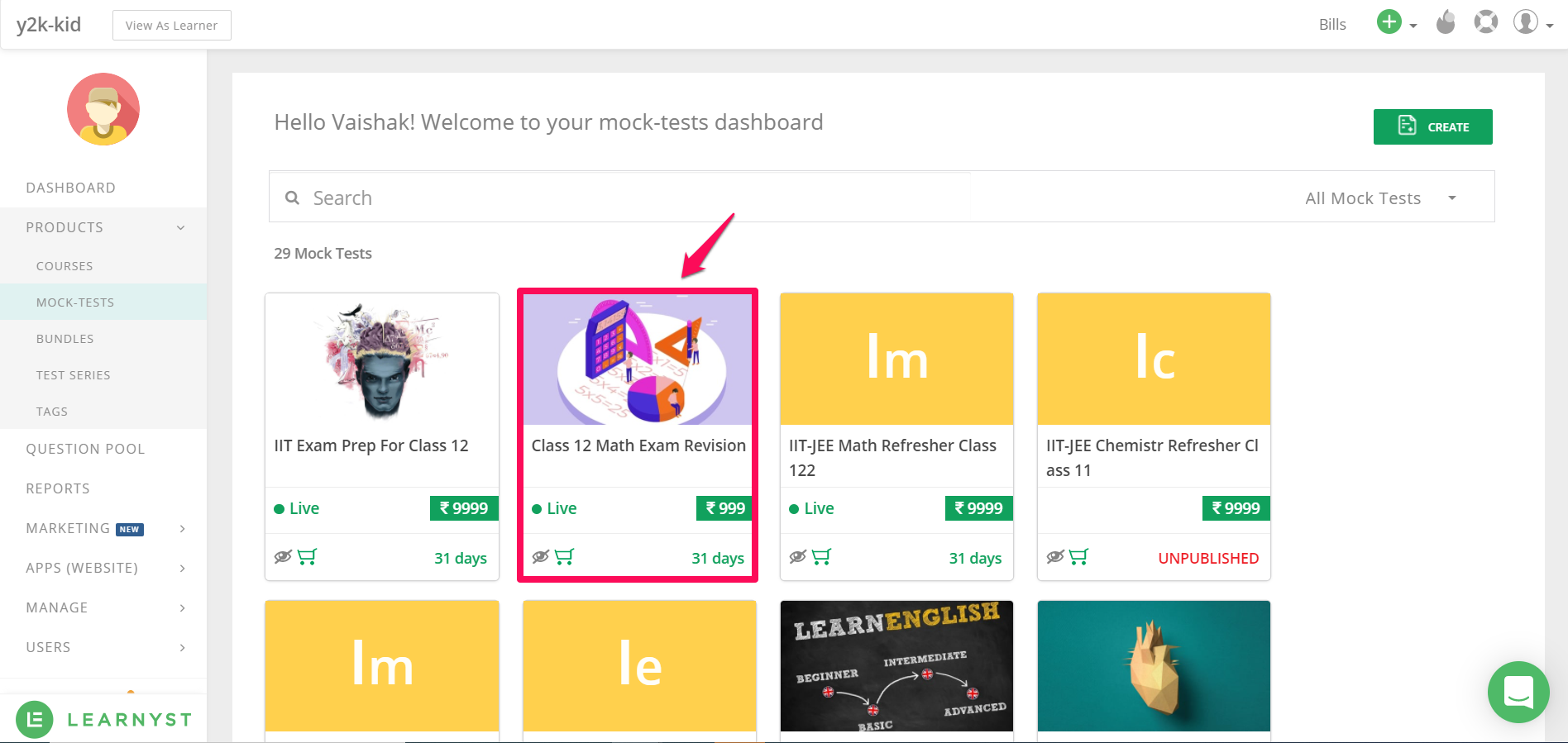This opens up the question builder. Next, Click on the MCQ question from the left panel.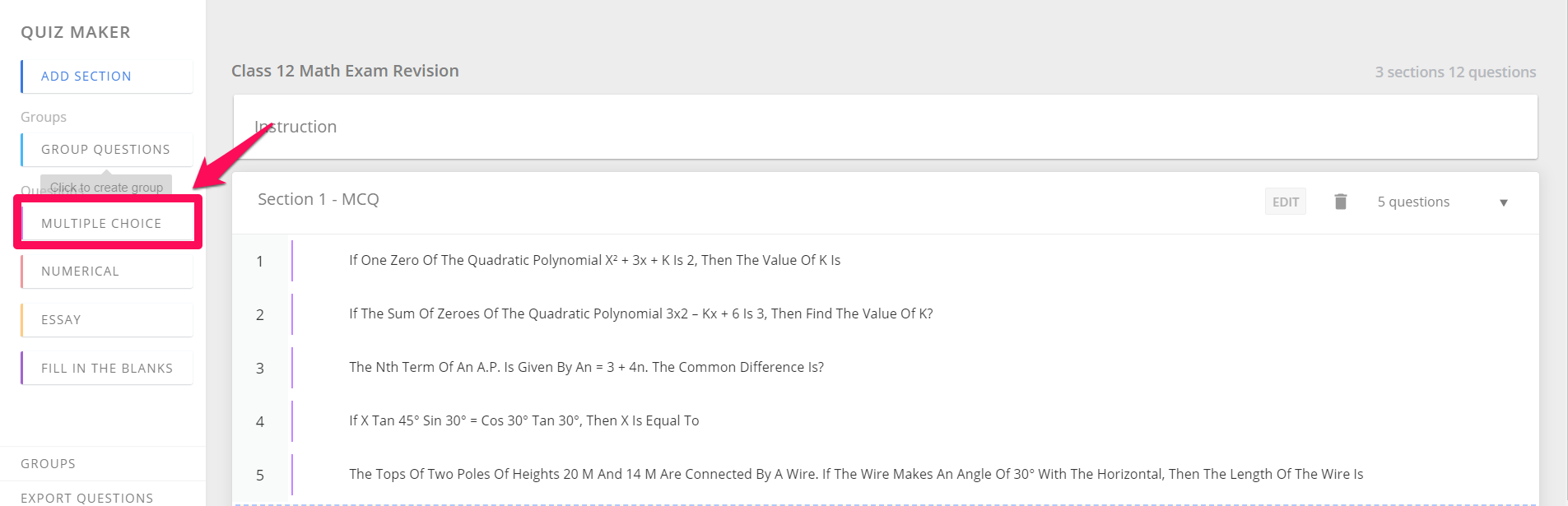Now, you can add the math equation to your question by selecting the math equation builder on the top right corner.### Related Article: How To Add Questions In Bulk Using Excel

This takes you to an interactive latex builder that looks like this.Let us see how to use this to enter our math equation:To enter 3 (x)^2, first enter the 3 in the latex editing window and select X^2 from the options as shown.Change the value of n to 2 and you will be able to see 3x^2 in the bottom.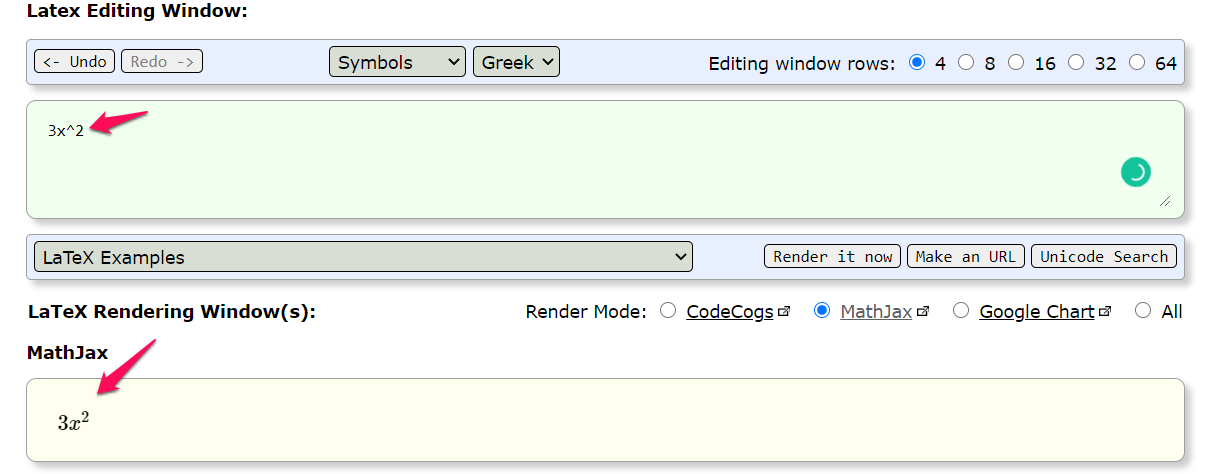Next, lets enter add -4sqrt(3)x in the equation

To do that, add -4 and select the sqrt function and 3 from the dropdown list as shown.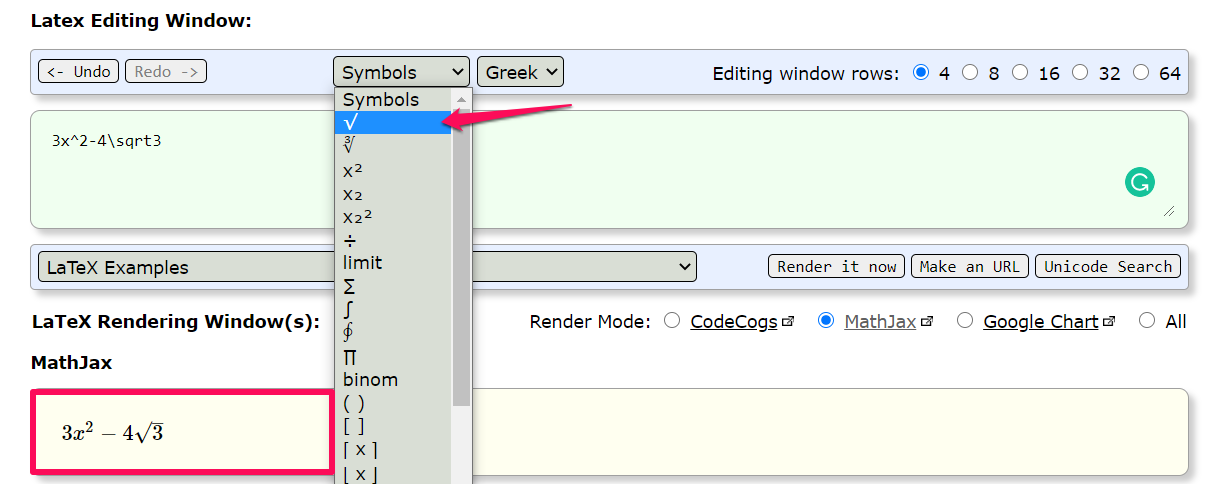Lastly, you can complete the equation by adding +4=0 as shown below.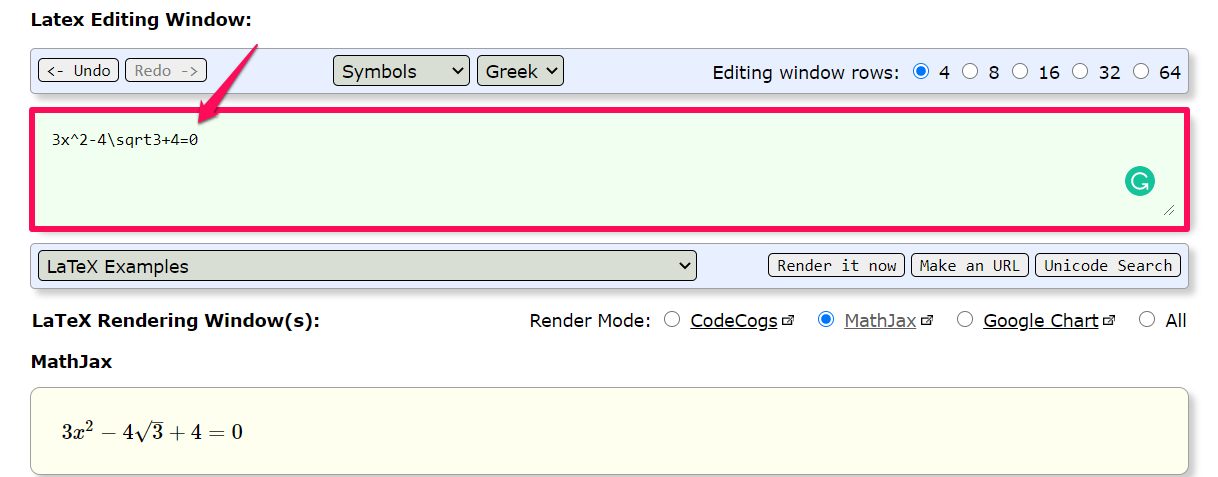For adding the math equation to our quiz, we need the equation written in the Latex editing window. The MathJax screen below just shows the output.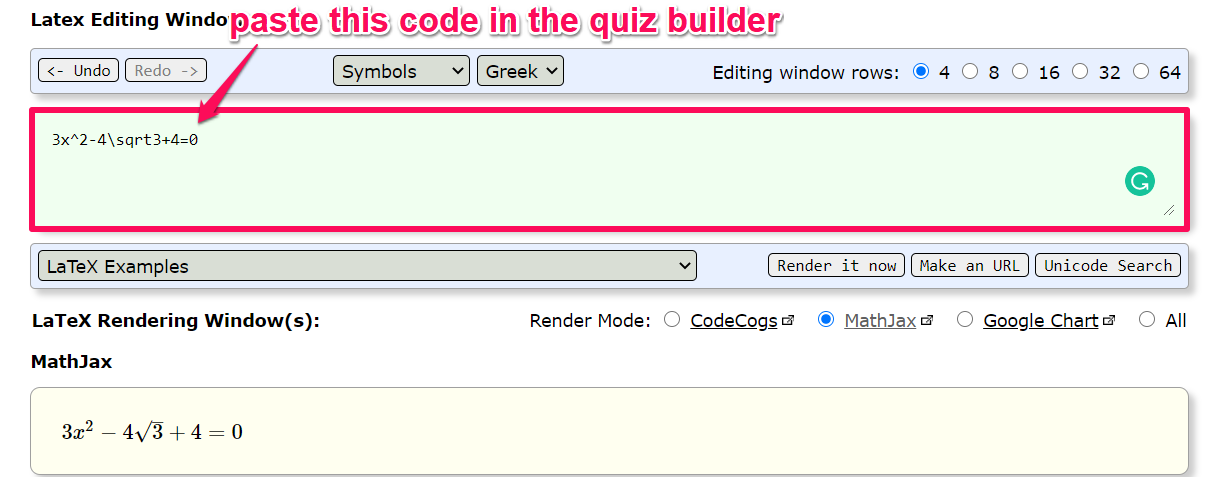Now, let us go to our question builder and paste this code. Here is how to do it.

After pasting the code in the question builder, I will put the equation within brackets like the below image.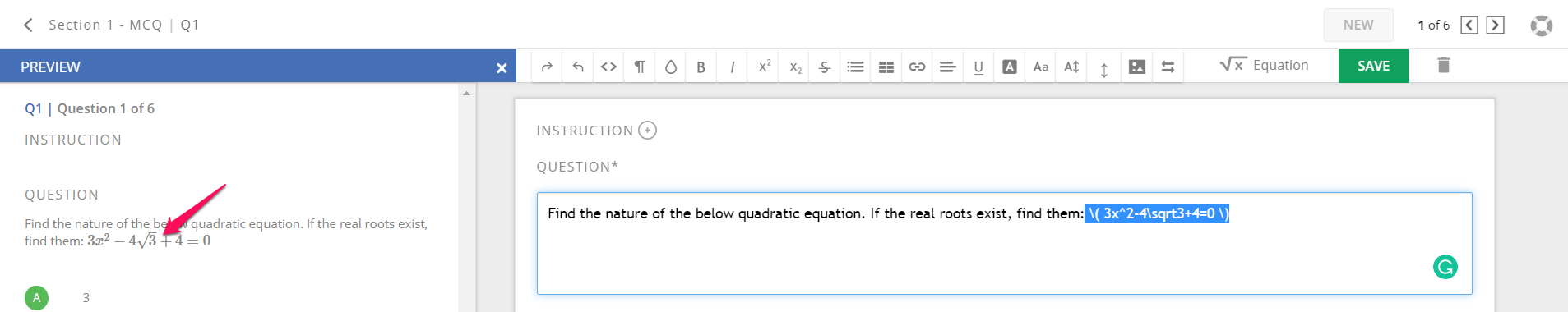Syntax is like this. Enter $$space your math equation space$$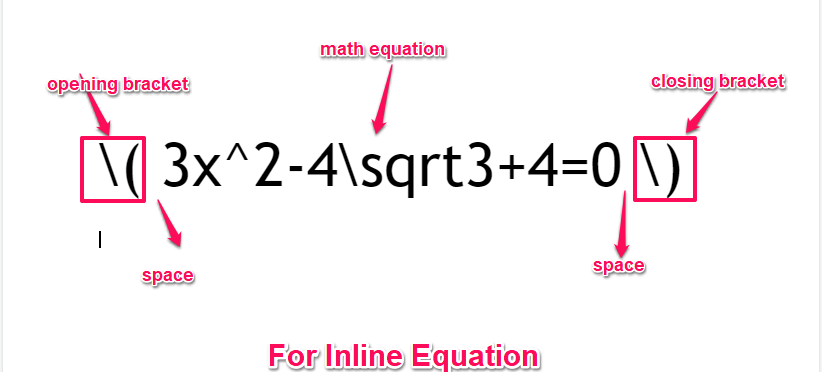Note: Use the preview option to get the preview of your equation.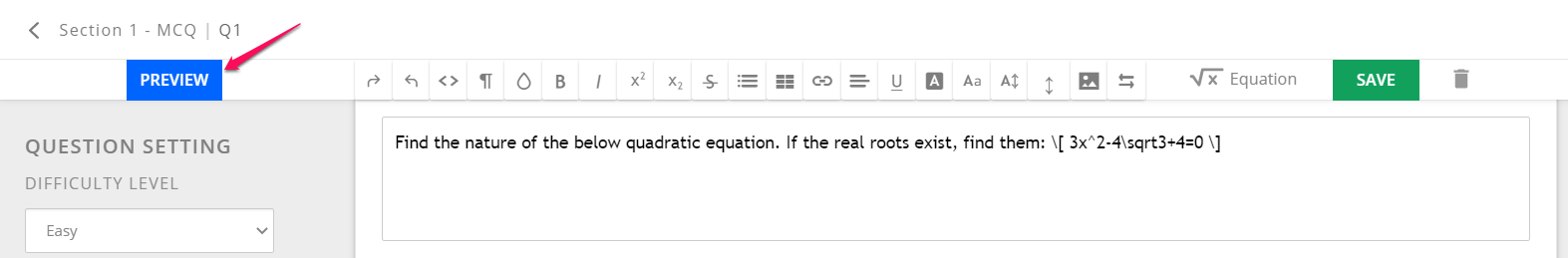So, if you want the equation to appear in the same line of text, known as the inline equation, you can use the circular brackets syntax.Now, if you want the equation to appear on a separate line, you can use the square brackets instead of circular ones. (The syntax will be the same)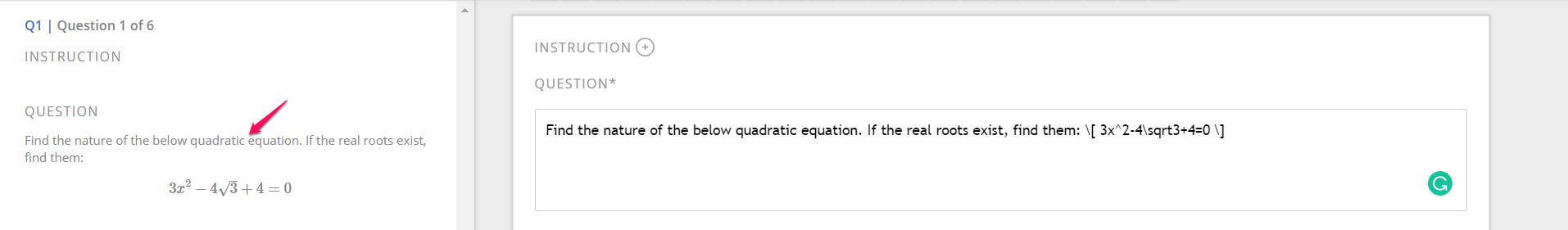As you can see the equation appears on a separate line. Refer the below syntax to construct your equation.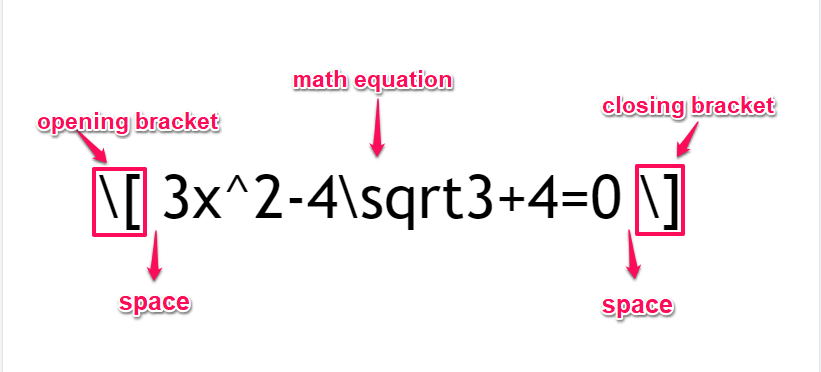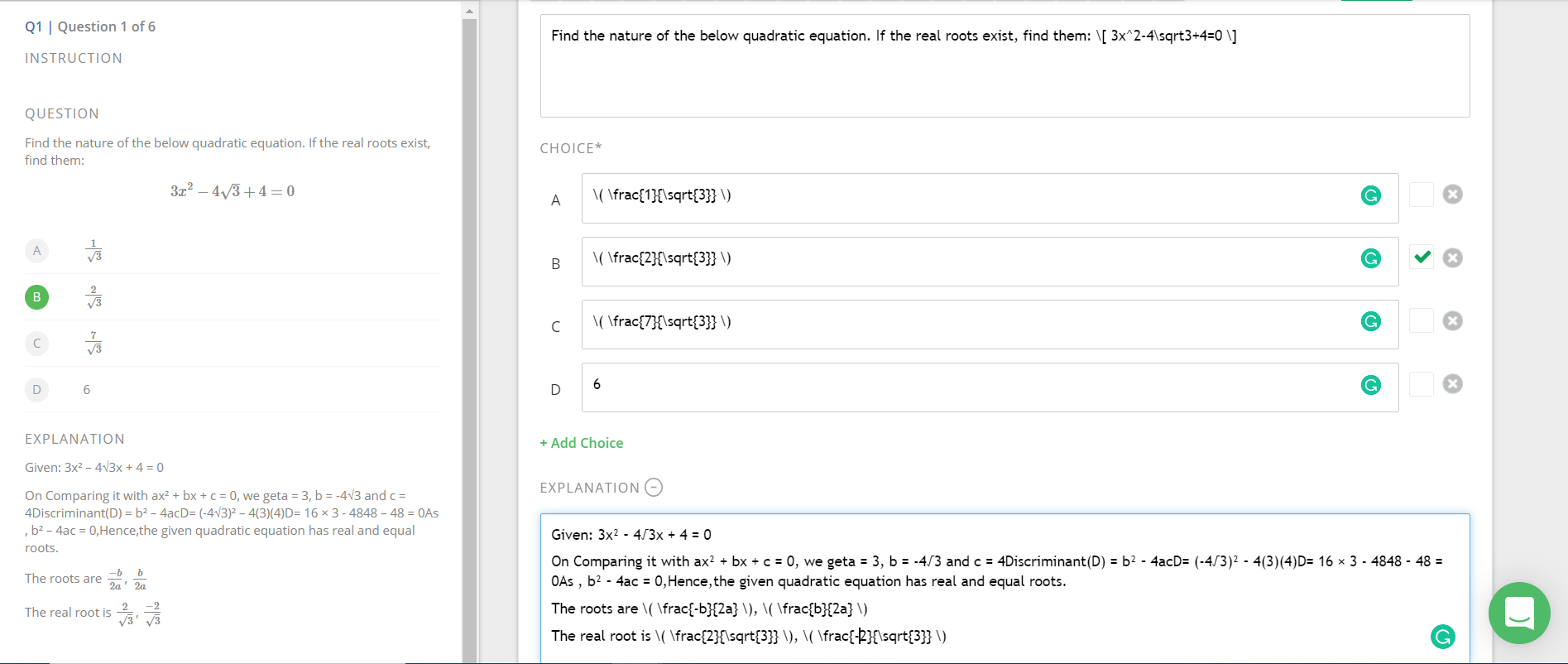### Related: How To Add Questions In Bulk Using Word

Once done with question creation click on the Save button to save changes to your multiple-choice question. Your students will be able to see the math equation.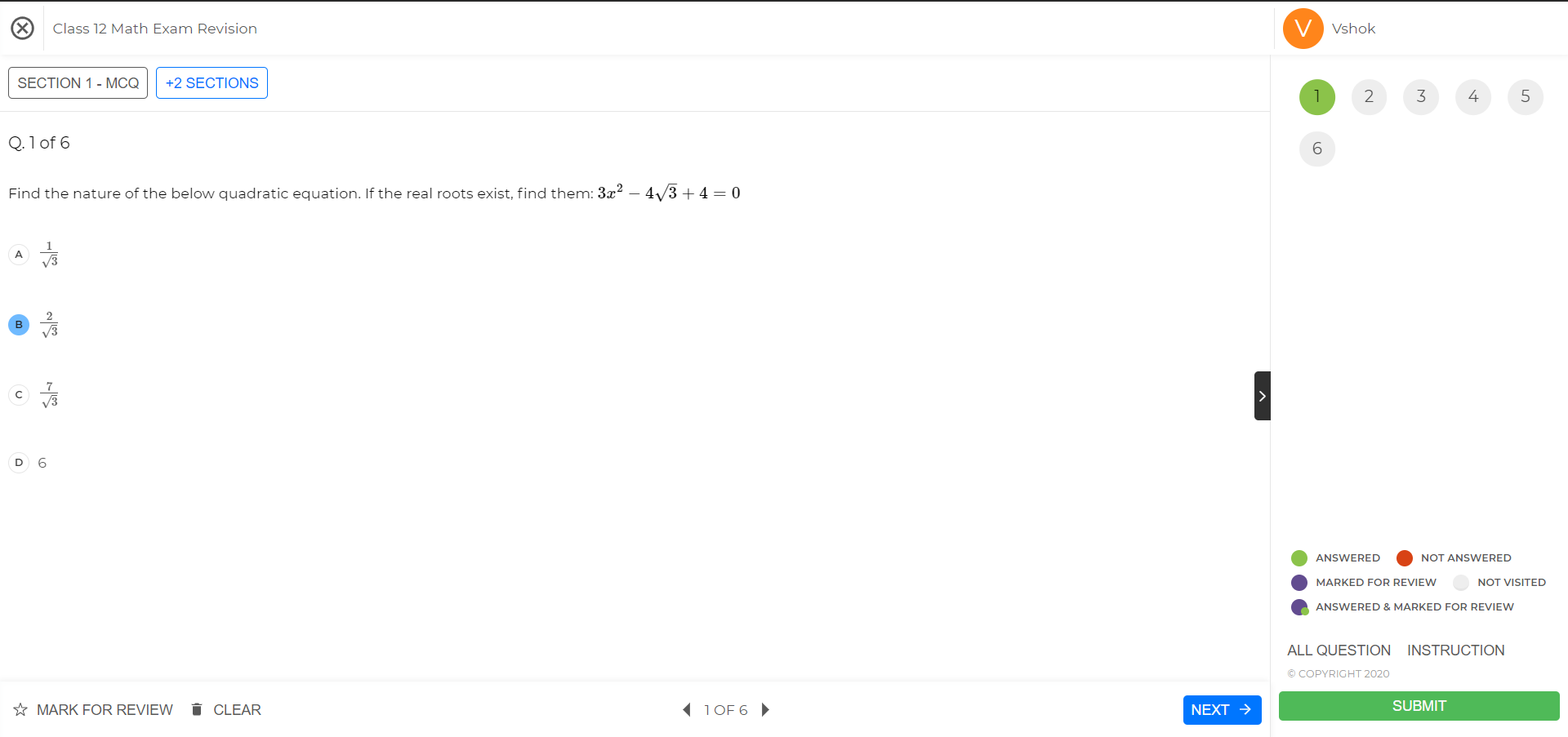I hope this support article, helped you to create and upload math equations in latex form.

To know how to bulk upload questions in bulk using templates, check our updated article on how to upload question using word template and how to upload questions using excel template.